# Symmetry

Eva loves symmetry in shapes and numbers. Yesterday she invented a completely new kind of symmetry - divisible symmetry. She wrote all five-digit numbers with different digits with the following property:
the first digit is divisible by 1, the second by 2, the third by 3, the fourth by 4 and the fifth by 5, regardless of whether the digit reads from left to right or from right to left.

List all five-digit numbers with Eva's divisible symmetry. How many are there?

n =  12

### Step-by-step explanation:

1. 50005
2. 50305
3. 50605
4. 50905
5. 54045
6. 54345
7. 54645
8. 54945
9. 58085
10. 58385
11. 58685
12. 58985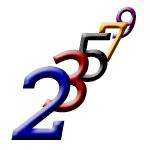Did you find an error or inaccuracy? Feel free to write us. Thank you!## Related math problems and questions:

• Four-digit numbers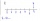Find four-digit numbers where all the digits are different. For numbers, the sum of the third and fourth digits is twice the sum of the first two digits, and the sum of the first and fourth digits is equal to the sum of the second and third digits. The di
• Divisible by nineHow many three-digit natural numbers in total are divisible without a remainder by the number 9?
• Seven-segmet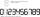Lenka is amused that he punched a calculator (seven-segment display) numbers and used only digits 2 to 9. Some numbers have the property that their image in the axial or central symmetry was again give some number. Determine the maximum number of three-di
• Five-digit numberAnna thinks of a five-digit number that is not divisible by three or four. If he increments each digit by one, it gets a five-digit number that is divisible by three. If he reduces each digit by one, he gets a five-digit number divisible by four. If it sw
• Divisibility by 12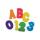Replace the letters A and B by digits so that the resulting number x is divisible by twelve /find all options/. x = 2A3B How many are the overall solutions?
• PIN codePIN on Michael credit card is a four-digit number. Michael told this to his friend: • It is a prime number - that is, a number greater than 1, which is only divisible by number one and by itself. • The first digit is larger than the second. • The second d
• Twenty-fiveHow many are three-digit natural numbers divisible by 25?
• Z9-I-4Kate thought a five-digit integer. She wrote the sum of this number and its half at the first line to the workbook. On the second line wrote a total of this number and its one fifth. On the third row, she wrote a sum of this number and its one nines. Fina
• 7 digit numberIf 3c54d10 is divisible by 330, what is the sum of c and d?
• What are 3What are the two digits which when inserted in the blank spaces will make 234 _ _ divisible by 8?
• By sixFrom the digits 1,2,3,4 we create the long integer number 123412341234. .. .. , which will have 962 digits. Is this number divisible by 6?
• A five-digit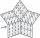A five-digit odd number has the sum of all digits (digits) five and contains two zeros. If we move each digit in the number one place to the left and move the first digit to the last place, we get a number 20,988 smaller. Find this unknown five-digit numb
• Sum of the digitsHow many are two-digit natural numbers that have the sum of the digits 9?
• Three-digit numbersHow many are all three-digit numbers that are made up of digits 0,2,5,7 and are divisible by 9 if the digits can be repeated?
• How many 4How many four-digit numbers that are divisible by ten can be created from the numbers 3, 5, 7, 8, 9, 0 such no digits repeats?
• Six-digit primesFind all six-digit prime numbers that contain each one of digits 1,2,4,5,7, and 8 just once. How many are they?
• Three digits numberFrom the numbers 1, 2, 3, 4, 5 create three-digit numbers that digits not repeat and number is divisible by 2. How many numbers are there?